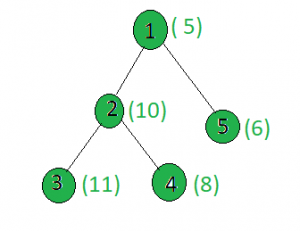# Count the nodes of the given tree whose weight has X as a factor

Given a tree, and the weights of all the nodes, the task is to count the nodes whose weights are divisible by x.

Examples:

Input:x = 5
Output: 2
Only the nodes 1 and 2 have weights divisible by 5.

## Recommended: Please try your approach on {IDE} first, before moving on to the solution.

Approach: Perform dfs on the tree and for every node, check if it’s weight is divisible by x or not. If yes then increment the count.

Below is the implementation of the above approach:

## C++

 `// C++ implementation of the approach ` `#include ` `using` `namespace` `std; ` ` `  `long` `ans = 0; ` `int` `x; ` `vector<``int``> graph; ` `vector<``int``> weight(100); ` ` `  `// Function to perform dfs ` `void` `dfs(``int` `node, ``int` `parent) ` `{ ` ` `  `    ``// If weight of the current node ` `    ``// is divisible by x ` `    ``if` `(weight[node] % x == 0) ` `        ``ans += 1; ` ` `  `    ``for` `(``int` `to : graph[node]) { ` `        ``if` `(to == parent) ` `            ``continue``; ` `        ``dfs(to, node); ` `    ``} ` `} ` ` `  `// Driver code ` `int` `main() ` `{ ` `    ``x = 5; ` ` `  `    ``// Weights of the node ` `    ``weight = 5; ` `    ``weight = 10; ` `    ``weight = 11; ` `    ``weight = 8; ` `    ``weight = 6; ` ` `  `    ``// Edges of the tree ` `    ``graph.push_back(2); ` `    ``graph.push_back(3); ` `    ``graph.push_back(4); ` `    ``graph.push_back(5); ` ` `  `    ``dfs(1, 1); ` ` `  `    ``cout << ans; ` ` `  `    ``return` `0; ` `} `

## Java

 `// Java implementation of the approach  ` `import` `java.util.*; ` ` `  `class` `GFG ` `{ ` `     `  `static` `long` `ans = ``0``;  ` `static` `int` `x;  ` `static` `Vector> graph=``new` `Vector>();  ` `static` `Vector weight=``new` `Vector();  ` ` `  `// Function to perform dfs  ` `static` `void` `dfs(``int` `node, ``int` `parent)  ` `{  ` ` `  `    ``// If weight of the current node  ` `    ``// is divisible by x  ` `    ``if` `(weight.get(node) % x == ``0``)  ` `        ``ans += ``1``;  ` ` `  `    ``for` `(``int` `i = ``0``; i < graph.get(node).size(); i++)  ` `    ``{  ` `        ``if` `(graph.get(node).get(i) == parent)  ` `            ``continue``;  ` `        ``dfs(graph.get(node).get(i), node);  ` `    ``}  ` `}  ` ` `  `// Driver code  ` `public` `static` `void` `main(String args[]) ` `{  ` `    ``x = ``5``;  ` ` `  `    ``// Weights of the node  ` `    ``weight.add(``0``);  ` `    ``weight.add(``5``);  ` `    ``weight.add(``10``);;  ` `    ``weight.add(``11``);;  ` `    ``weight.add(``8``);  ` `    ``weight.add(``6``);  ` `     `  `    ``for``(``int` `i = ``0``; i < ``100``; i++) ` `    ``graph.add(``new` `Vector()); ` ` `  `    ``// Edges of the tree  ` `    ``graph.get(``1``).add(``2``);  ` `    ``graph.get(``2``).add(``3``);  ` `    ``graph.get(``2``).add(``4``);  ` `    ``graph.get(``1``).add(``5``);  ` ` `  `    ``dfs(``1``, ``1``);  ` ` `  `    ``System.out.println(ans);  ` `} ` `}  ` ` `  `// This code is contributed by Arnab Kundu `

## C#

 `// C# implementation of the approach ` `using` `System; ` `using` `System.Collections.Generic; ` ` `  `class` `GFG ` `{ ` `     `  `static` `long` `ans = 0;  ` `static` `int` `x;  ` `static` `List> graph = ``new` `List>();  ` `static` `List<``int``> weight = ``new` `List<``int``>();  ` ` `  `// Function to perform dfs  ` `static` `void` `dfs(``int` `node, ``int` `parent)  ` `{  ` ` `  `    ``// If weight of the current node  ` `    ``// is divisible by x  ` `    ``if` `(weight[node] % x == 0)  ` `        ``ans += 1;  ` ` `  `    ``for` `(``int` `i = 0; i < graph[node].Count; i++)  ` `    ``{  ` `        ``if` `(graph[node][i] == parent)  ` `            ``continue``;  ` `        ``dfs(graph[node][i], node);  ` `    ``}  ` `}  ` ` `  `// Driver code  ` `public` `static` `void` `Main(String []args) ` `{  ` `    ``x = 5;  ` ` `  `    ``// Weights of the node  ` `    ``weight.Add(0);  ` `    ``weight.Add(5);  ` `    ``weight.Add(10);;  ` `    ``weight.Add(11);;  ` `    ``weight.Add(8);  ` `    ``weight.Add(6);  ` `     `  `    ``for``(``int` `i = 0; i < 100; i++) ` `    ``graph.Add(``new` `List<``int``>()); ` ` `  `    ``// Edges of the tree  ` `    ``graph.Add(2);  ` `    ``graph.Add(3);  ` `    ``graph.Add(4);  ` `    ``graph.Add(5);  ` ` `  `    ``dfs(1, 1);  ` ` `  `    ``Console.WriteLine(ans);  ` `} ` `} ` ` `  `// This code contributed by Rajput-Ji `

Output:

```2
```

My Personal Notes arrow_drop_upIf you like GeeksforGeeks and would like to contribute, you can also write an article using contribute.geeksforgeeks.org or mail your article to contribute@geeksforgeeks.org. See your article appearing on the GeeksforGeeks main page and help other Geeks.

Please Improve this article if you find anything incorrect by clicking on the "Improve Article" button below.

Improved By : andrew1234, Rajput-Ji

Article Tags :
Practice Tags :

Be the First to upvote.

Please write to us at contribute@geeksforgeeks.org to report any issue with the above content.